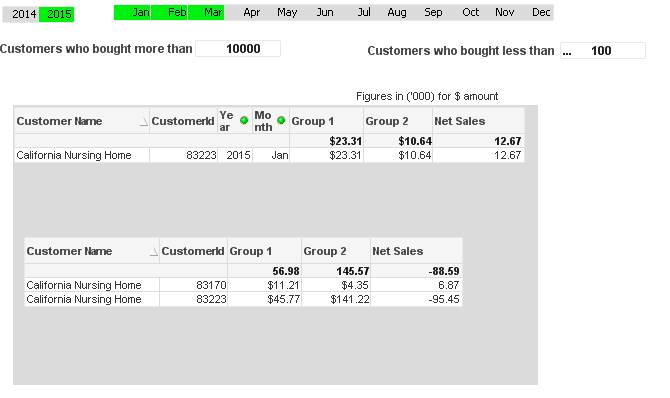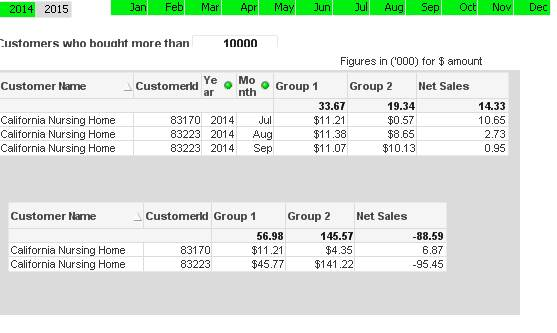# New to QlikView

Discussion board where members can get started with QlikView.

Announcements
Save the Date: QlikWorld Online, June 24-25, 2020. Free global virtual event for data integration and data analytic gurus. Register Today
HighlightedNot applicable

## Expression in Alternate States

Dear QV Experts,

In a straight table, need to display five columns,

CustomerID, CustomerName, Group1Sales , Group2Sales , NetSales [Group1Sales - Group2Sales].

Here Group1Sales & Group2Sales are two alternate states.

In the screenshot below,

If I include the Year & Month column in the Straight Table, it displays correctly if I select Year & Month List Boxes [ which is not part of alternate states].

But when I remove the Year & Month Columns in Straight Table, it calculates the sales for all the years & months for the customer.

Selected 2015 & Month Jan, Feb,Mar . The first straight table shows the correct values.Second Example.

If Year 2014 & All the months selected, Then still the second straight table displays the same value as before.Expression for Group1:

if(
If(Metrics='Dollar',
num(sum({[yes]<[CustomerSales] = {">=\$(=vInputAmtMore)"}>} CustomerSales )/1000,'\$#,##0.00'),
if(Metrics='Case',
num(sum({[yes]<[CustomerCases] = {">=\$(=vInputAmtMore)"}>} CustomerCases ),'#,##0.00'),
if(Metrics='Each',
num(sum({[yes]<[CustomerEach] = {">=\$(=vInputAmtMore)"}>} CustomerEach ),'#,##0.00')
)))>=
\$(=(vInputAmtMore/1000)),
If(Metrics='Dollar',
num(sum({[yes]<[CustomerSales] = {">=\$(=vInputAmtMore)"}>} CustomerSales )/1000,'\$#,##0.00'),
if(Metrics='Case',
num(sum({[yes]<[CustomerCases] = {">=\$(=vInputAmtMore)"}>} CustomerCases ),'#,##0.00'),
if(Metrics='Each',
num(sum({[yes]<[CustomerEach] = {">=\$(=vInputAmtMore)"}>} CustomerEach ),'#,##0.00')
))),)

Please guide / suggest me to get the correct values in Group1 Sales if Year & Month not included in the Straight Table also.

THanks,

Sasi

7 Replies
HighlightedMVP

## Re: Expression in Alternate States

I assume that [yes] is the name of your alternate state for Group1?

=If(
If(Only({[yes]} Metrics) = 'Dollar',
Num(Sum({[yes]<[CustomerSales] = {">=\$(=vInputAmtMore)"}>} CustomerSales)/1000,'\$#,##0.00'),
If(Only({[yes]} Metrics) = 'Case',
Num(Sum({[yes]<[CustomerCases] = {">=\$(=vInputAmtMore)"}>} CustomerCases ),'#,##0.00'),
If(Only({[yes]} Metrics) = 'Each',
Num(Sum({[yes]<[CustomerEach] = {">=\$(=vInputAmtMore)"}>} CustomerEach ),'#,##0.00')
)))>=\$(=(vInputAmtMore/1000)),
If(Only({[yes]} Metrics) = 'Dollar',
Num(Sum({[yes]<[CustomerSales] = {">=\$(=vInputAmtMore)"}>} CustomerSales)/1000,'\$#,##0.00'),
If(Only({[yes]} Metrics) = 'Case',
Num(Sum({[yes]<[CustomerCases] = {">=\$(=vInputAmtMore)"}>} CustomerCases ),'#,##0.00'),
If(Only({[yes]} Metrics) = 'Each',
Num(Sum({[yes]<[CustomerEach] = {">=\$(=vInputAmtMore)"}>} CustomerEach
),'#,##0.00')
))),)

you might need to update the state in your variable vInputAmtMore if it is a expression also.

HighlightedNot applicable

## Re: Expression in Alternate States

Hi Sunny,

Yeah Correct. [yes] & [no] is the alternate states for Group1 & Group2.

But only Year & Month is not part of the Alternate States. Because based on the requirement, we should not have

two List Boxes for Year & Month.

Can anyone suggest me how to integrate the impacts of selection of year & month in the alternate states.

Thanks a lot,

Sasi

HighlightedMVP

## Re: Expression in Alternate States

First I want to point out an additional comma (,) at the end of the expression. Seems like a typo, but make sure you fix that part. For the expression with selection of Year and Month selection from inherited state, try this:

=If(
If(Only({[yes]}*{1<Year = \$::Year, Month = \$::Month>} Metrics) = 'Dollar',
Num(Sum({[yes]<[CustomerSales] = {">=\$(=vInputAmtMore)"}*{1<Year = \$::Year, Month = \$::Month>}>} CustomerSales)/1000,'\$#,##0.00'),
If(Only({[yes]}*{1<Year = \$::Year, Month = \$::Month>} Metrics) = 'Case',
Num(Sum({[yes]<[CustomerCases] = {">=\$(=vInputAmtMore)"}*{1<Year = \$::Year, Month = \$::Month>}>} CustomerCases ),'#,##0.00'),
If(Only({[yes]}*{1<Year = \$::Year, Month = \$::Month>} Metrics) = 'Each',
Num(Sum({[yes]<[CustomerEach] = {">=\$(=vInputAmtMore)"}*{1<Year = \$::Year, Month = \$::Month>}>} CustomerEach ),'#,##0.00')
)))>=\$(=(vInputAmtMore/1000)),
If(Only({[yes]}*{1<Year = \$::Year, Month = \$::Month>} Metrics) = 'Dollar',
Num(Sum({[yes]<[CustomerSales] = {">=\$(=vInputAmtMore)"}*{1<Year = \$::Year, Month = \$::Month>}>} CustomerSales)/1000,'\$#,##0.00'),
If(Only({[yes]}*{1<Year = \$::Year, Month = \$::Month>} Metrics) = 'Case',
Num(Sum({[yes]<[CustomerCases] = {">=\$(=vInputAmtMore)"}*{1<Year = \$::Year, Month = \$::Month>}>} CustomerCases ),'#,##0.00'),
If(Only({[yes]}*{1<Year = \$::Year, Month = \$::Month>} Metrics) = 'Each',
Num(Sum({[yes]<[CustomerEach] = {">=\$(=vInputAmtMore)"}*{1<Year = \$::Year, Month = \$::Month>}>} CustomerEach
),'#,##0.00')
))),)

HighlightedNot applicable

## Re: Expression in Alternate States

Hi Sunny,

Still it is not working. it is displaying -

HighlightedNot applicable

## Re: Expression in Alternate States

Hi Sunny,HighlightedNot applicable

## Re: Expression in Alternate States

Hi Sunny,

Small changes I have done from your end,

=if(

If(Metrics='Dollar',

num(sum({[yes]<Year= \$::Year,Month = \$::Month,[CustomerSales] = {">=\$(=vInputAmtMore)"}>} CustomerSales )/1000,'\$#,##0.00'),

if(Metrics='Case',

num(sum({[yes]<Year= \$::Year,Month = \$::Month,[CustomerCases] = {">=\$(=vInputAmtMore)"}>} CustomerCases ),'#,##0.00'),

if(Metrics='Each',

num(sum({[yes]<Year= \$::Year,Month = \$::Month,[CustomerEach] = {">=\$(=vInputAmtMore)"}>} CustomerEach ),'#,##0.00')

)))>=\$(=(vInputAmtMore/1000)),

If(Metrics='Dollar',

num(sum({[yes]<Year= \$::Year,Month = \$::Month,[CustomerSales] = {">=\$(=vInputAmtMore)"}>} CustomerSales )/1000,'\$#,##0.00'),

if(Metrics='Case',

num(sum({[yes]<Year= \$::Year,Month = \$::Month,[CustomerCases] = {">=\$(=vInputAmtMore)"}>} CustomerCases ),'#,##0.00'),

if(Metrics='Each',

num(sum({[yes]<Year= \$::Year,Month = \$::Month,[CustomerEach] = {">=\$(=vInputAmtMore)"}>} CustomerEach ),'#,##0.00')

))),)

HighlightedMVP

## Re: Expression in Alternate States

Awesome.

Thanks for sharing this. I knew I was close, but couldn't think of the correct syntax.